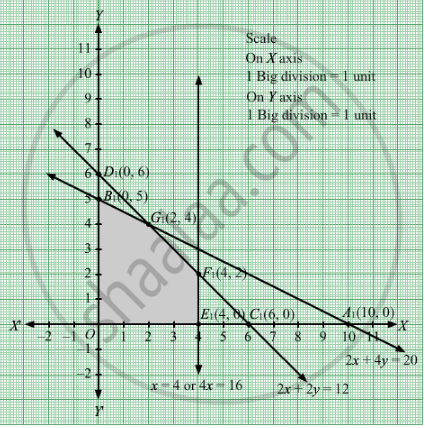# A Factory Uses Three Different Resources for the Manufacture of Two Different Products, 20 Units of the Resources A, 12 Units of B and 16 Units of C Being Available - Mathematics

Sum

A factory uses three different resources for the manufacture of two different products, 20 units of the resources A, 12 units of B and 16 units of C being available. 1 unit of the first product requires 2, 2 and 4 units of the respective resources and 1 unit of the second product requires 4, 2 and 0 units of respective resources. It is known that the first product gives a profit of 2 monetary units per unit and the second 3. Formulate the linear programming problem. How many units of each product should be manufactured for maximizing the profit? Solve it graphically.

#### Solution

Let x units of first product and y units of second product be manufactured.
Therefore, $x, y \geq 0$

The given information can be tabulated as follows:

 Product Resource A Resource B Resource C First(x) 2 2 4 Second(y) 4 2 0 Availability 20 12 16

Therefore, the constraints are

$2x + 4y \leq 20$

$2x + 2y \leq 12$

$4x + 0y \leq 16 \text{ or } 4x \leq 16$

It is known that the first product gives a profit of 2 monetary units per unit and the second 3. Therefore, profit gained from x units of first product and y units of second product is 2x monetary units and 4y monetary units respectively.

Total profit = Z =  $2x + 3y$ which is to be maximised
Thus, the mathematical formulat​ion of the given linear programmimg problem is
Max Z =   $2x + 3y$
subject to

$2x + 4y \leq 20$
$2x + 2y \leq 12$
$4x + 0y \leq 16 \text { or} 4x \leq 16$

$x, y \geq 0$

First we will convert inequations into equations as follows :
2x + 4y = 20, 2x + 2y = 12, 4x = 16, x = 0 and y = 0

Region represented by 2x + 4y ≤ 20:
The line 2x + 4y = 20 meets the coordinate axes at A1(10, 0) and B1(0, 5) respectively. By joining these points we obtain the line 2x + 4y = 20. Clearly (0,0) satisfies the 3x + 2y = 210. So,the region which contains the origin represents the solution set of the inequation 2x + 4y ≤ 20.

Region represented by 2x + 2y ≤ 12:
The line 2x +2y =16 meets the coordinate axes at C1(6, 0) and D1(0, 6) respectively. By joining these points we obtain the line 2x + 2y = 12. Clearly (0,0) satisfies the inequation 2x + 2y ≤ 12. So, the region which contains the origin represents the solution set of the inequation 2x + 2y ≤ 12.
Region represented by 4x ≤ 16:
The line 4x =16 or x = 4 is the line passing through the point E1(4, 0) and is parallel to axis.The region to the left of the line x = 4 would satisfy the inequation 4x ≤ 16.
Region represented by x ≥ 0 and y ≥ 0:
Since, every point in the first quadrant satisfies these inequations. So, the first quadrant is the region represented by the inequations x ≥ 0, and ≥ 0.
The feasible region determined by the system of constraints 2x + 4y ≤ 20, 2x + 2y ≤ 12, 4x ≤ 16, x ≥ 0 and y ≥ 0 are as followsThe corner points are O(0, 0), B1(0, 5), G1 $\left( 2, 4 \right)$ F1(4,  2) and E1(4, 0).

The values of Z at these corner points are as follows

 Corner point Z= 2x + 3y O 0 B1 15 G1 16 F1 14 E1 8

The maximum value of Z is 16 which is attained at G1 $\left( 2, 4 \right)$ Thus, the maximum profit is 16 monetary units obtained when 2 units of first product and 4 units of second product were manufacture .
Concept: Graphical Method of Solving Linear Programming Problems
Is there an error in this question or solution?

#### APPEARS IN

RD Sharma Class 12 Maths
Chapter 30 Linear programming
Exercise 30.4 | Q 14 | Page 52